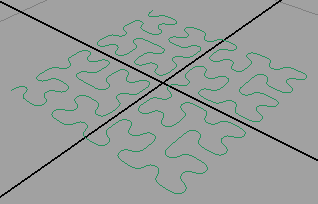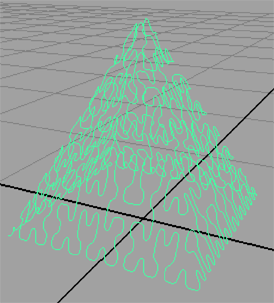### Hilbert CurveMel Implementation

#### Introduction

This tutorial follows on from the introduction to Hilbert curves, "Hilbert Curve: Concepts & Implementation". The purpose of this tutorial is to develop examples of Hilbert curves for use with Maya. Figure 1 shows the output of the mel script given in listing 1.Figure 1

In the `hilbert` proc the y coordinate is maintained at zero, hence the curve is aligned to the x-z plane. However, some fairly interesting effects can be achieved by assigning a non-zero value to the y coordinate of the control vertices (cv's). The next section explores a few examples of "lofted" Hilbert curves.

Listing 1 (hilbert.mel)

 ```vector \$cvs[]; global proc hilbert(float \$x0, float \$y0, float \$xi, float \$xj, float \$yi, float \$yj, int \$n) { global vector \$cvs[]; if(\$n <= 0) { float \$x = \$x0 + (\$xi + \$yi)/2; float \$z = \$y0 + (\$xj + \$yj)/2; // Output the coordinates of the cv \$cvs[size(\$cvs)] = <<\$x,0,\$z>>; } else { hilbert(\$x0, \$y0, \$yi/2, \$yj/2, \$xi/2, \$xj/2, \$n-1); hilbert(\$x0+\$xi/2, \$y0 + \$xj/2, \$xi/2, \$xj/2, \$yi/2, \$yj/2, \$n-1); hilbert(\$x0+\$xi/2+\$yi/2,\$y0+\$xj/2+\$yj/2,\$xi/2, \$xj/2, \$yi/2, \$yj/2, \$n-1); hilbert(\$x0+\$xi/2+\$yi, \$y0+\$xj/2+\$yj, -\$yi/2,-\$yj/2,-\$xi/2,-\$xj/2, \$n-1); } } global proc drawHilbert(int \$degree, int \$reps) { global vector \$cvs[]; // Cleanup from the previous run clear \$cvs; // Delete curves from prior runs of the script string \$items[] = `ls "curve*"`; if(size(\$items) > 0) { select \$items; delete; } // Begin the Mel curve statement string \$cmd = "curve -d " + \$degree + " -periodic 0 "; // Calculate the cv's hilbert(-0.5, -0.5 , 1.0, 0.0, 0.0, 1.0, \$reps); // Copy the cv data for(\$n = 0; \$n < size(\$cvs); \$n++) \$cmd += " -p " + \$cvs[\$n]; // Create the curve eval(\$cmd); } drawHilbert 3 4; ```

#### Variations of the Hilbert Curve

Setting a value for the y coordinate based on absolute difference between the x and z coordinates of a cv, as shown in the following code snippet, generates a pyramid - figure 2.

 ```if(\$n <= 0) { float \$x = \$x0 + (\$xi + \$yi)/2; float \$z = \$y0 + (\$xj + \$yj)/2; float \$y = 0; if(abs(\$z) > abs(\$x)) \$y = 0.5 - abs(\$z); else \$y = 0.5 - abs(\$x); \$y *= 2; // Output the coordinates of the cv \$cvs[size(\$cvs)] = <<\$x,\$y,\$z>>; }```Figure 2 - Pyramid

 The next variation applies a similiar test to the absolute values of x and z but the result is quite different - figure 3.

 ```if(\$n <= 0) { float \$x = \$x0 + (\$xi + \$yi)/2; float \$z = \$y0 + (\$xj + \$yj)/2; float \$y = 0; if(abs(\$z) < abs(\$x)) \$y = 0.5 - abs(\$z); else \$y = 0.5 - abs(\$x); \$y *= 2; // Output the coordinates of the cv \$cvs[size(\$cvs)] = <<\$x,\$y,\$z>>; }```Figure 3 - "roof"

 By setting the y coordinate proportional to the distance of the cv to the origin of the curve a "conical" shape can be produced - figure 4.

 ```if(\$n <= 0) { float \$x = \$x0 + (\$xi + \$yi)/2; float \$z = \$y0 + (\$xj + \$yj)/2; float \$y = 0; \$y = 0.5 - mag(<<\$x,\$y,\$z>>); \$y *= 1.5; // Output the coordinates of the cv \$cvs[size(\$cvs)] = <<\$x,\$y,\$z>>; }```Figure 4 - Conical

© 2002- Malcolm Kesson. All rights reserved.